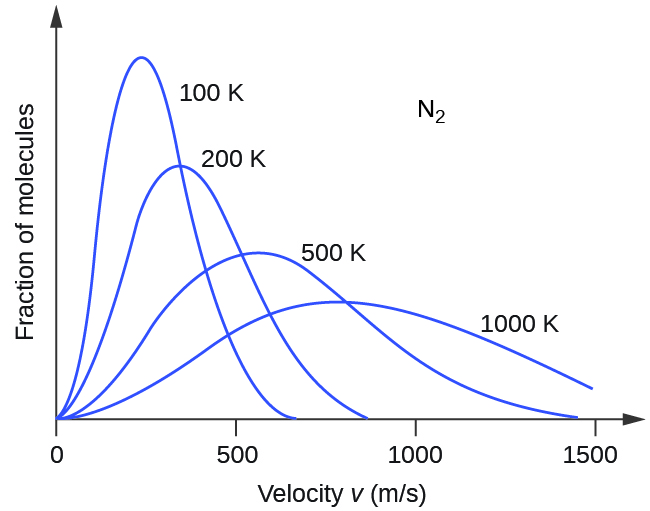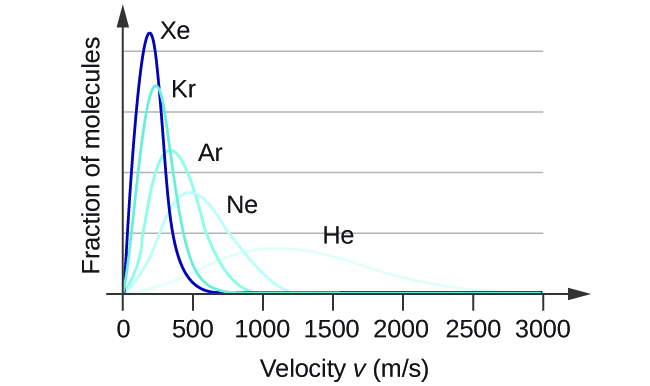# 9.5 The kinetic-molecular theory  (Page 3/6)

 Page 3 / 6

If the temperature of a gas increases, its KE avg increases, more molecules have higher speeds and fewer molecules have lower speeds, and the distribution shifts toward higher speeds overall, that is, to the right. If temperature decreases, KE avg decreases, more molecules have lower speeds and fewer molecules have higher speeds, and the distribution shifts toward lower speeds overall, that is, to the left. This behavior is illustrated for nitrogen gas in [link] .The molecular speed distribution for nitrogen gas (N 2 ) shifts to the right and flattens as the temperature increases; it shifts to the left and heightens as the temperature decreases.

At a given temperature, all gases have the same KE avg for their molecules. Gases composed of lighter molecules have more high-speed particles and a higher u rms , with a speed distribution that peaks at relatively higher velocities. Gases consisting of heavier molecules have more low-speed particles, a lower u rms , and a speed distribution that peaks at relatively lower velocities. This trend is demonstrated by the data for a series of noble gases shown in [link] .Molecular velocity is directly related to molecular mass. At a given temperature, lighter molecules move faster on average than heavier molecules.

## The kinetic-molecular theory explains the behavior of gases, part ii

According to Graham’s law, the molecules of a gas are in rapid motion and the molecules themselves are small. The average distance between the molecules of a gas is large compared to the size of the molecules. As a consequence, gas molecules can move past each other easily and diffuse at relatively fast rates.

The rate of effusion of a gas depends directly on the (average) speed of its molecules:

$\text{effusion rate}\phantom{\rule{0.2em}{0ex}}\propto \phantom{\rule{0.2em}{0ex}}{u}_{\text{rms}}$

Using this relation, and the equation relating molecular speed to mass, Graham’s law may be easily derived as shown here:

${u}_{\text{rms}}=\sqrt{\frac{3RT}{m}}$
$m=\phantom{\rule{0.2em}{0ex}}\frac{3RT}{{u}_{\text{r}\text{m}\text{s}}^{2}}\phantom{\rule{0.2em}{0ex}}=\phantom{\rule{0.2em}{0ex}}\frac{3RT}{{\overline{u}}^{2}}$
$\frac{\text{effusion rate A}}{\text{effusion rate B}}\phantom{\rule{0.2em}{0ex}}=\phantom{\rule{0.2em}{0ex}}\frac{{u}_{\text{r}\text{m}\text{s}\phantom{\rule{0.2em}{0ex}}\text{A}}}{{u}_{\text{r}\text{m}\text{s}\phantom{\rule{0.2em}{0ex}}\text{B}}}\phantom{\rule{0.2em}{0ex}}=\phantom{\rule{0.2em}{0ex}}\frac{\sqrt{\frac{3RT}{{m}_{\text{A}}}}}{\sqrt{\frac{3RT}{{m}_{\text{B}}}}}\phantom{\rule{0.2em}{0ex}}=\phantom{\rule{0.2em}{0ex}}\sqrt{\frac{{m}_{\text{B}}}{{m}_{\text{A}}}}$

The ratio of the rates of effusion is thus derived to be inversely proportional to the ratio of the square roots of their masses. This is the same relation observed experimentally and expressed as Graham’s law.

## Key concepts and summary

The kinetic molecular theory is a simple but very effective model that effectively explains ideal gas behavior. The theory assumes that gases consist of widely separated molecules of negligible volume that are in constant motion, colliding elastically with one another and the walls of their container with average velocities determined by their absolute temperatures. The individual molecules of a gas exhibit a range of velocities, the distribution of these velocities being dependent on the temperature of the gas and the mass of its molecules.

## Key equations

• ${u}_{\text{r}\text{m}\text{s}}=\sqrt{\overline{{u}^{2}}}=\sqrt{\frac{{u}_{1}^{2}+{u}_{2}^{2}+{u}_{3}^{2}+{u}_{4}^{2}+\dots }{n}}$
• ${\text{KE}}_{\text{avg}}=\phantom{\rule{0.2em}{0ex}}\frac{3}{2}RT$
• ${u}_{\text{rms}}=\sqrt{\frac{3RT}{m}}$

How does an element differ from a compound? How are they similar?
an element is an indivisible particles that can take part in a reaction and consist of smaller or tiny particles i.e proton, neutrons and electron while a compound is when two or more element chemically combine together. They are similar when they are homogeneous compound. they take the same rxn.
Yusuf
How to get the Lewis formula of SeCl+3
hi,I'm new here can I join the conversation
EZEA
what is the structural formula for starch
Starch is a mixture (of chemicals) of amylose and amylopectin. Both are macromolecules and polymers. You can search on wikipedia.
Abdelkarim
what is the roles of filter bed
Fathmat
what is the roles of Alu m
Fathmat
what is the roles of chlorine
Fathmat
Roles can be classified or correlate it to different areas: For example: Chlorine can be used in reactions (in industry) to manufacture HCl, which then can be used for other things. Or in swimming pools to kill bacteria. Or as a component in compounds with pharmaceutical roles (drugs). For Al:
Abdelkarim
Its dentisty value is suitable to be used in alloys (mixture of metals) in aircraft bodies. Also, Aluminium foils, Tin cans,.. Some of them are also in Al overhead cables in streets and long roads.
Abdelkarim
what is chemistry
Maxamed
what is the meaning of exceedingly
it is an adverb which means extremely
Rohini
what is atomic chemistry?
Lewis structure for no3
Lewis structure for no3
what is weak acid
It is an acid which partially ionises in water.
Abdelkarim
what is incandescence
Clifton
what makes it glow
Clifton
why is it red, irange and yellow in color
Clifton
hello am new here and I want to join you
Aliyu
hello
Clifton
hi
Aliyu
too
Gillian
hello i am new here please i want to join this group
Paul
Hi, I'm also new here
Salaudeen
Hi
Keeya
hello guys !!
Sourav
what is pressure?
The force applied to suction Area of the body
Ahmed
Matter composed of exceedingly small paticle called atom.
Yushao
questions related to metals
occurrence and preparation of the representatives metals
Regina
list the 20, periodic table and their symbols
hydrogen:h helium;he lithium:l beryllium:be Boron:b Carbon;C Nitrogen:n Oxygen:O FLUORINE:f Neon:n Sodium:s Magnesium:mg Aluminum:a Silicon:s Phosphorus:p Sulphur:s Chlorine:c Argon;a Potassium:p Calcium:c
Benita
Hydrogen, helium, lithium, beryllium, boron, carbon, nitrogen, oxygen, fluorine, neon, sodium, magnesium, aluminium, silicon, phosphorus, sulphur, chlorine, argon, potassium, calcium
Cudjoe
what is a solute
Any substance that is disolved in a liqid solvent to create a solution
Fifa
sorry liquid
Fifa
it's a liquid substance
Fathmat
hello group
Ayomide
is the substance that dissolves in the solvent
Amos
so is HCl ionic compound
No, covalent compound ➡️ molecule. As both H and Cl are non-metals and and form covalent bind by sharing valence e-. But can fully ionice in water forming H+ (a proton, a reason for acidity) and Cl- (anion =Chloride) Hydrogen Chloride is a gas at room; Hydrochloric acid = HCl (aq), dissolved in w
Abdelkarim
Form covalenr bond*
Abdelkarim
The question marks are an emoji in the first sentence is an unread emoji. HCl Covalent compund -> molecule
Abdelkarim
Hi.
Queen
Hi
Calvin
Yh
Cudjoe
yes
Amos
what is chemistry
is the study of composition of substances and the way they behave under different conditions
Amos
how do calculate n1 though n6 any help on understanding the concept
Clifton
where can I get the test bank or mcqs ? any idea ?
what are the types of intermolecular forces between organic compounds
Intermolecular forces exist between molecules of different units like van der waal force, hydrogen bonds
Salaudeen
What is chemistry
scientific study of structure of substances and of the way that they react with other substances
Haider
Thanks
khausar
welcome
Haider
Hi
khausar
hi 2
Haider
whr u from
Haider
are u writing GCE
Equin
Cameroon and u
Equin
hello
DanielByByBy Brooke DelaneyBy Donyea SweetsBy JavaChamp TeamBy OpenStaxBy OpenStaxBy Cath YuBy Maureen MillerBy RhodesBy David GeltnerBy Stephanie Redfern